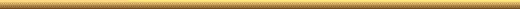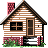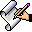Here is the Transcription of Mr. Milan Sulc's presentation from his vedio

Numbers have two characteristics, cardinal and ordinal.

The cardinal characteristic gives it the nature of counting whereas the ordinal number gives the order or rank of a number as a composite or prime

A composite number may be divided into smaller numbers thus it is a partitioning of the number into smaller numbers whereas a prime may be divided only by 1 or itself. Their property is a property independent of bases and independent of space and time.

E.g.

 Order Prime Copm 1 2 4 2 3 6 3 5 8 4 7 9 5 11 10 6 13 12

A positional partitional number PPN is a number that may be the sum of a number and its index in the prime or composite domain.

E.g. 8= 3 + 5 where the 3rd prime is 5

8= 2 +6 where the 2nd composite is 6

This is another way of looking at the construction of a number from a cardinal and its associated ordinal.

There are mathematical methods to determine the divisibility of a number but no mathematical method exists to determine the rank of a composite or prime. In other words the order of any number must be found by counting.

There are no mathematical methods to determine a PPN. Tables must be constructed in order to do so. PPNs may be constructed in several ways and so to determine the rank of such a PPN firstly all the possible tables or ways of constructing a number (eg P-C=C; P+C=C; P+C=P , P+R=P etc, where P is prime, C is composite and R is rank) must be made up to the desired value of the PPN and the PPN located in one of the tables. So the process of construction of a number is very intricate and complicated and no mathematical methods exist and tabulation is the only way.

The PPN and number properties have a significant function in the Quran in positioning the verses in the Quran. The PPNs may be tabulated as tables of sums of composites or differences of composites and their order or as tables of differences of composites or primes and their order.

Case in point how many verses do Sura Al Tawba or sura 9 have. Is it 127 verses or 129 verses? As per Hadith each verse in the Quran is witnessed by 2 persons but verse 128 and 129 was witnessed by one person. This is a historical account.

So case in question is where does sura 9 end? At 9:127 or at 9:129

9:127 is the 1362nd numbered verse in the Quran and the 670 un-initialled verse in the Quran. 1143rd composite is 1362 and 9 x 127 = 1143. The concatenation of 9 and 127 to form 9127 can only occur in the decimal system, it would be different say n binary form whereas 9 x 127 = 1143 is a base independent property and this property hold in any base system. Thus God confirms in a base independent way that Sura 9 has 127 verses.

Thus 9:128 is the 1363rd numbered verse and the 671st un-initialled verse and 9:128 is then 1364th numbered verse and the 672nd un-initialled verse. Now 1364 is a PPN and is the 207th such PPN where Prime - Composite = Composite and may be written as:

1364= 1697(265th prime) - 333(265th composite)

In this case Subordinate Rank, SR=207 and Mutual Rank, MR=265 is the common or mutual rank of the prime and composite.

If we take the verses 9:128 and 9:129 to be part of the Quran then the 1364th verse is 9:129.

The Quran says over it is 19 and sura 19 has 98 verses.

The 1998th ayah in the Quran inclusive of 9:128 and 9:129 is 16:97.

1364= 1697(265th prime) - 333(265th composite)

This would confirm 9:129

549th composite is 671 and 549 + 671 gives 1220.

1220th ayah in the Quran is 8:60

And 6:71 is the 860th ayah in the Quran

Also 671 = 11 x 61 and 1161 = 9 x 129

This fixes the position of 9:129 but only showing nothing is against the will of GOD and that the Design of GOD is that this ayah would be inserted at that position.

(This is what Mr. Sulc is trying to prove of GOD's signs that 9:127 is the true count and that 9:128 and 9:129 are not from GOD but had to be added only in that position or in other words we cannot even decide our transgression.)

Going back to :

1364 is a PPN and is the 207th such PPN where Prime - Composite = Composite and may be written as:

207. 1364 = 1697(265th prime) - 333(265th composite)

In this case Subordinate Rank, SR=207 and Mutual Rank, MR=265 is the common or mutual rank of the prime and composite.

We have the following: 208th composite is 265 and 208 + 265 = 473 = 11x43

1364 - 207=1157; 1157 is the 1143rd such PPN.

333+265 is C+R=598 and the rank is 214. 214 + 598 = 812 and 812 is the 670th composite and 9:127 is the 670th un-initialled verse.

333 written in base 19 is

in base 10: 3x 19 raised to the power of 2+3x19 raised to the power of 2 + 3x19 raised to the power of 0= 3 x 361 + 3 x 19 + 3 = 1143

Since 8th prime is 19 then 1143 written in base 8 is in base 10: 1x8 raised to the power of 3 + 1x 8 raised to the power of 2 +4x8 raised to the power of 0=611

219. 271 + 340 = 611

C + R =C

Here 219 is the subordinated rank of the rank of a composite +composite giving a composite.

271st composite is 340

340th Ayah in the Quran is the 333rd initialled verse in the Quran and is 3:47. (Here Milan misquotes it as 3:33 instead of the 333rd initialled verse being 3:47)

219 + 611 = 830

830+684=1514

684th composite is 830.

1514th ayah in the Quran witnessed by two person i.e. excluding 9:128 and 9:129 is 11:43

1514 is the rank of the PPN 1532.

1532nd ayah in the Quran is 11:61 witnessed by 2 persons(9:128 and 9:129 is excluded here). So although 11x 61 points to 9129 but the fact that 11:61 is witnessed by 2 persons point to 9127.

1273rd composite is 1514 and 9:127 has the 1273rd mention of GOD.

1273rd PPN is 1288.

1288th ayah in the Quran is 9:53

953 composite is 1143.

As mentioned earlier 1514th PPN is 1532.

1532nd composite is 1813

1813th ayah is

15:13 15:13 is the 1143rd initialled ayah.

1272nd composite is 1513

1272nd ayah is 9:37

9 x 37= 333 we saw earlier that 333 written in base 19 is 1143 in base 10.

159th prime is 937

159th composite is 206

206 + 937 = 1143

15:9 We have indeed revealed this reminder and We will surely be its guardian.

1143 - 159 = 984

98:4 is where the 19th mention of Al Bayyinah is found (The proof)

817th composite is 984.

817 + 984 = 1801 1801st ayah is 15:1

15:1 is the 1131st initialled ayah

1131st prime is 9127

36th prime is 151

36 + 151 = 187

187th prime is 1117

1117th PPN is 1131; 1131 prime is 9127

187 = 11 x 17

[8:17] Therefore, you did not kill them; on the contrary, GOD1031 had already damned them. And you did not throw, when you threw, it was GOD1032 who threw. And this is in order to test the faithful with the best of tests. Indeed GOD1033 is All Hearing All Knowing

This ayah shows GOD is in control

120th composite is 158

120th prime is 659

158 + 659 = 817

817 + 120 = 937

And as seen earlier 9 x 37 = 333 and 333 written in base 19 is 1143 in base 10 and 1143=9x127.

All these underlying mathematical structures of cardinals and ordinals bring as back to 9127.

As seen earlier 15:13 is the 1813th ayah and the 1143rd initialled ayah.

1813th composite is 2135

1575. 1813 + 2135 = 3948

where 1575 is the subordinate rank.

3948 + 1575 = 5523

5523rd ayah is 74:30(witnessed by two persons)

1697 + 7430 = 9127

1697 was pointing to 9129 but when added to the verifier 7430 gives 9127.

5523 is a composite formed from the subordinated rank and its composite as shown above and this is the 1349 the such composite.

5523 - 1349 = 4174

And [4:174] O you people, indeed a proof has come to you from your Lord, and We have revealed to you a proven enlightenment.

1575 = 1303 + 272

Where the mutual rank of the prime and composite is 213.

1575 - 213 = 1362

And 1362nd verse is 9:127

9127 is a reversible prime meaning that 7219 is a prime too.

This is the 213th reversible prime.

From above was shown 1801.

279th composite is 350

279th prime is 1801

1801 - 350 = 1451

1451 - 279 = 1172 where 279 is the mutual rank. The subordinate rank of this composite is 231.

1172nd ayah is 8:12

670th composite is 812. 670th un-initialled ayah is 9:127

1172 - 231 = 941

941st composite is 1131

1131st prime is 9127

350 + 279 = 629

514th composite is 629

629 + 514 = 1143 = 9x127

[2:79] Therefore, woe to those who write the scripture with their hand and say this is from GOD35 in order to obtain a cheap material gain. Woe to them for what they wrote and woe to them for what they gained

So it clearly points out that 9:128 and 9:129 are fabrications.

231st ayah is 2:224

862. 2224 = 1211 + 1013

1013th composite is 1211

Where 862 is the subordinate rank.

2224 - 862 =1362 and 1362nd verse is 9:127

12:11 is the 1605th ayah

1352nd composite is 1605

1352nd PPN is 1352

The number of verses including the uncounted Bismillah up to 9:127 is 1369

Some people have objected to the concatenation of numbers.

473 = 11 x 43 473rd verse from the end of the Quran is 80:6

806 =13 x 62 giving concatenation 1362

1143 composite is 1362

This shows that GOD is aware of concatenation.

1129th partionable composite is 1362 and

1129 = 622 + 507

507th composite is 622

The 507th verse from the end of the Quran including the uncounted Bismillah is 80:6.

Thus GOD says:

[72:28] This is in order for Him to know that they have delivered all their Lord's messages and He fully encompasses everything they do and He has counted the number of all things

When GOD has counted all things numerically it implies both cardinally and ordinally as shown in the examples.P.O. Box. 251902.. Los Angeles, .CA.. 90025